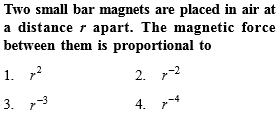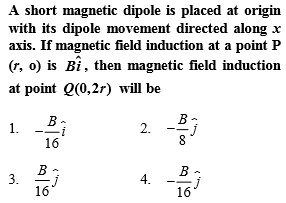Complete Question Bank + Test Series
Complete Question Bank

Difficulty Level:Complete Question Bank + Test Series
Complete Question Bank

Difficulty Level:

A magnet of magnetic moment 20 C.G.S. units is freely suspended in a uniform magnetic field of intensity 0.3 C.G.S. units. The amount of work done in deflecting it by an angle of ${30}^{o}$ in C.G.S. units is
(a) 6

(b) $3\sqrt{3}$

(c) $3\left(2-\sqrt{3}\right)$

(d) 3

Complete Question Bank + Test Series
Complete Question Bank

Difficulty Level:

The magnetic field due to a short magnet at a point on its axis at distance X cm from the middle point of the magnet is 200 Gauss. The magnetic field at a point on the neutral axis at a distance X cm from the middle of the magnet is
(a) 100 Gauss               (b) 400 Gauss
(c) 50 Gauss                 (d) 200 Gauss

Complete Question Bank + Test Series
Complete Question Bank

Difficulty Level:

A magnet of magnetic moment  is placed along the x-axis in a magnetic field $\stackrel{\to }{B}=\left(0.5\stackrel{^}{i}+3.0\stackrel{^}{j}\right)T$. The torque acting on the magnet is

(a) 175 $\stackrel{^}{k}$ N-m

(b) 150 $\stackrel{^}{k}$ N-m

(c) 75 $\stackrel{^}{k}$ N-m

(d) 25$\sqrt{37}$ $\stackrel{^}{k}$ N-m